Buffer Effects <---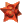Back---> 2D Effects

# Wildfire`s Color EffectsThis operator adds or subtracts the rgb-components of two images.

• Alpha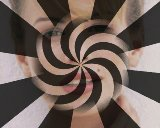This operator modifies the brightness of an image using an alpha-channel.

• AntiqueThis operator turns the colors of an image to look "anitique".

• BalancingThis operator allows you modify the colors of an image using different methods.This operator allows you to modify image-colors smoothly. It features all methods of the Balancing PlugIn. But instead of applying a fixed set of parameters to the whole image a smooth gradient (parallel or radial) is created and applied. Animate the Values for nice animations.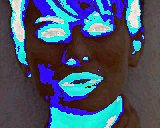Using this effect you can mathematical AND, OR and EOR the single bits of all points with an bit-mask which you can specify.

• ColorRegionThis operator detects a region of similar colors around a specified centre. It may be used for creating Alpha-Channels.

• ColorToGrayThis operator converts an image into grayscale.

• Convolve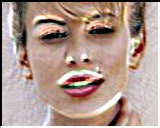This operator applies a 3x3- or 5x5 convolution-matrix to an image.

• EdgeDetect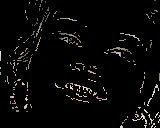This operator uses an advanced algorithm to detect edges within an image. In contrary commonly applied methods (e.g. a simple convolution-matrix) the detected edges are left in original colors. Furthermore, "weak edges" can be suppressed adjusting the contrast-value.

• EmbossThis operator converts an image in a gray relief.

• HSLThis operator allows you to modify the colors in HSL-colorspace ((Red, Green, Blue)->(Hue, Saturation, Luminosity)).

• LineArtThis operator applies the well-known LineArt-effect to an image.

• LUTThis operator applies a LookUpTable to an image. Unlike to all other image-processors LUT's are represented by Envelopes and can be morphed ! There are 6 Envelopes: a Start- and an End-Envelope for every component. After specifying the number of frames the Start-Envelope is morphed into the End-Envelope. Different point-counts are possible. On the X-axis you see the `normal` color and on the y-axis you see the `changed` color. -If your envelope is a line from 0,0 to 255,255 then all colors stay unchanged. -If your envelope is a line from 0,255 to 255,0 then you have negative colors!

• MathColorThis is a quite complex (and slow) effect. For every single image you can calculate the R,G and B values of every pixel seperatly. The formulas may contain the following symbols: - x,y ... x-and y coordinate of the current point - width ... width of the image - height ... height of the image - r,g,b ... colors of the curren point - e, pi ... constant mathematical values The left upper edge has the coordinates 1,1 and the right lower edge has the coordinates width, height. Examples: R=B=G=0 ... black image R=B=G=255 ... white image R=B=0, G=255 ... green image R=B=0, G=X ... green transition in x-direction R=B=0, G=sin(x/32) X width ... two green bars R=B=0, G=g+100 ... Balancing with green=100 R=B=0, G=sin(sqrt((width/2-x)^2+(height/2-y)^2) X 0.25) X 128+128 A wave around the image-centre.

• MedianFilterThis operator applies a median-filter to the image. (In the most cases there will be no visible change but the image will be smoother.)

• Negative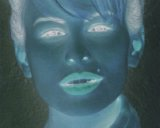This operator turns the colors of an image into negative ones. If you use this operator with an step of 2 then you`ll get a fast `flashing`.

• NegBright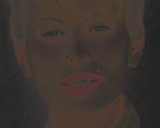This operator negates the brightness of an image. Use Step=2 for a nice flashing.

• NeonThis operator adds a glow effect to an image. Animate the radius and the tolerance values for nice effects.

• Noise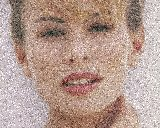This operator applies random noise to an image. Animate the intensity value for brighter/darker noise on your image.

• OilTransfer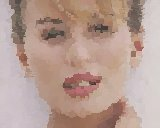This operator applies an OilTransfer-algorithm to an image. The result will look like drawn with oil-color.

• PosteriseThis operator reduces the number of bits per byte for every pixel of the image. The less bits you use the more `unreal` the result will look like.

• ReplaceComp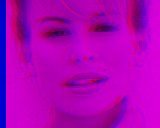This operator replaces a color-channel of an image with the red-channel of an other image. The main purpose of this PlugIn is to create Stereo-3D-Images.

• RIPThis operator removes all isolated (single) pixels from an image.

• SwapRGB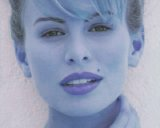This operator swaps the color-channels of an image. This looks strange on some images.

• Threshold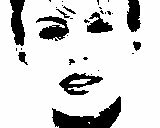This operator converts an image into black and white using a grayscale-level, this may be especially useful for the creation of alpha-channels.

Buffer Effects <---Back---> 2D Effects